# Double and dual numbers

(diff) ← Older revision | Latest revision (diff) | Newer revision → (diff)
Jump to: navigation, search

Hypercomplex numbers of the form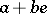, whereandare real numbers, and where the double numbers satisfy the relation, while the dual numbers satisfy the relation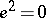(cf. Hypercomplex number). Addition of double and dual numbers is defined by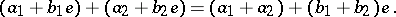Multiplication of double numbers is defined by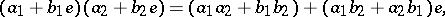and that of dual numbers byComplex numbers, double numbers and dual numbers are also called complex numbers of hyperbolic, elliptic and parabolic types, respectively. These numbers are sometimes used to represent motions in the three-dimensional spaces of Lobachevskii, Riemann and Euclid (see, for instance, Helical calculus).

Both double and dual numbers form two-dimensional (with base 1 and) associative-commutative algebras over the field of real numbers. As distinct from the field of complex numbers, these algebras comprise zero divisors, all these having the form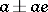in the algebra of double numbers. The algebra of double numbers may be split into a direct sum of two real number fields. Hence yet another name for double numbers — splitting complex numbers. Double numbers have yet another appellation — paracomplex numbers. The algebra of dual numbers is considered not only over the fieldof real numbers, but also over an arbitrary field or commutative ring. Letbe a commutative ring and letbe an-module. The direct sum of-modules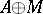equipped with the multiplication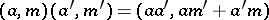is a commutative-algebra and is denoted by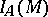. It is known as the algebra of dual numbers with respect to the module. The-moduleis identical with the ideal of the algebrawhich is the kernel of the augmentation homomorphism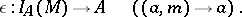The square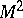of this ideal is zero, while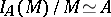. Ifis a regular ring the converse is also true: Ifis an-algebra andis an ideal insuch thatand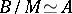, then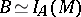, whereis regarded as an-module .

If, the algebra(then denoted by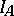) is isomorphic to the quotient algebra of the algebra of polynomialsby the ideal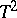. Many properties of an-module may be formulated as properties of the algebra; as a result, many problems on-modules can be reduced to corresponding problems in the theory of rings .

Letbe an arbitrary-algebra, letbe a homomorphism and let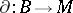be a derivation (cf. Derivation in a ring) ofwith values in the-module, regarded as a-module with respect to the homomorphism. The mapping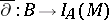(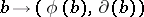) will then be a homomorphism of-algebras. Conversely, for any homomorphism of-algebras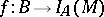the composition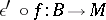, where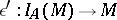is the projection ofonto, is an-derivation ofwith values in, regarded as a-module with respect to the homomorphism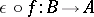. This property of double and dual numbers is utilized for the description of the tangent space to an arbitrary functor in the category of schemes , .

How to Cite This Entry:
Double and dual numbers. Encyclopedia of Mathematics. URL: http://encyclopediaofmath.org/index.php?title=Double_and_dual_numbers&oldid=46769
This article was adapted from an original article by I.V. Dolgachev (originator), which appeared in Encyclopedia of Mathematics - ISBN 1402006098. See original article